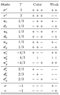# Grand Unification Theory fine structure constant

Orion1

I am inquiring if anyone here is qualified to numerically demonstrate the solution to this equation?

The equation is the Proton lifetime derived from the SU(5) Georgi-Glashow model listed in reference 1, eq. (19).

$$\tau_p \geq \frac{1}{\alpha_{(5)}^2} \frac{M_X^4}{m_p^5}$$

$$\tau_p \geq 10^{30} \; \text{years}$$

According to reference 1, the parameters are:
$$m_p \geq 0.9382 \; \text{GeV}$$ - Proton mass
$$M_X \geq 10^{14} \; \text{GeV}$$ - X Boson mass
$$\alpha_{(5)} = \; \text{?}$$ - SU(5) fine structure consant

Experimentally observed values:
$$\tau_p \geq 10^{32} \; \text{years}$$ - (1990)
$$\tau_p \geq 10^{35} \; \text{years}$$ - Super-Kamiokande

References for the symbolic mathematical proof to this equation and the value of $$\alpha_{(5)}$$ would be appreciated.

Reference:
http://en.wikipedia.org/wiki/Georgi-Glashow_model" [Broken]
http://en.wikipedia.org/wiki/Proton_decay" [Broken]
http://en.wikipedia.org/wiki/Electronuclear_force" [Broken]
http://en.wikipedia.org/wiki/Grand_unification_theory#cite_note-0"
http://hyperphysics.phy-astr.gsu.edu/hbase/forces/unify.html" [Broken]
http://hyperphysics.phy-astr.gsu.edu/hbase/astro/unify.html#c1"

Last edited by a moderator:

Homework Helper
Gold Member
2022 Award
I THINK that $$\alpha _U \approx \frac{1}{42}$$

source, particle physics by martin & shaw, page 269

BenTheMan
Well ordinary SU(5) is dead. You have to add matter, which in turn changes the prediction for proton decay. See papers by Pavel Perez, who loves non-SUSY GUTs.

Either way, the value of the SU(5) coupling constant is generally taken to be the place where the gauge couplings all sort of meet, which is (I think) 1/42 ish. The exact number is difficult to uncover because the agreement with unification is so poor. Look at Figure 15.1 in this review: http://pdg.lbl.gov/2007/reviews/gutsrpp.pdf. The way it works is that you take the place where $$\alpha_2 = \alpha_1$$, and call that unification. Then you add a threshold effect to the strong coupling constant to make it work. The threshold effect comes from new states at the GUT scale (generally 10^16 GeV or so) that begin to contribute to the beta functions. The problem is that the threshold effect has to be large, and it is hard to imagine how such a large contribution can come into save you. What Perez showed is that you CAN get such contributions, but the manner in which you get them seems to be rather inelegant.

If you're talking about SUSY GUTs, then SU(5) is STILL dead by super-K bounds. This was shown in a paper by Hitoshi Murayama and Aaron Pierce.

In fact, the only viable GUTs are the non-minimal ones, in which you add a lot of stuff to make it work. Even SUSY SO(10) still needs to be dressed up with abelian family symmetries and such.

Finally, you should be careful. In the SUSY GUT case, you have proton decay operators coming in at dimension 5, which comes from spartner exchange, and can be a real pain in the ass.

Last edited:
Orion1

$$\alpha_{(5)} = \alpha _U \approx \frac{1}{42}$$ - SU(5) fine structure constant
$$m_p = 0.9382720298 \; \text{GeV}$$ - Proton mass
$$m_X = 10^{14} \; \text{Gev}$$ - SU(5) - X Boson mass
$$m_X = 4.32037202924731 \cdot 10^{16} \; \text{GeV}$$ - Super-Kamiokande X baryon mass

$$\boxed{\tau_p = \frac{\hbar m_X^4}{10^9 e m_p^5 \alpha_{U}}}$$

$$\boxed{\tau_p = 3.80164285275096 \cdot 10^{33} \; \text{s} \; \; \; (1.20549304057298 \cdot 10^{26} \; \; \text{years})}$$ - SU(5) Proton decay lifetime:

$$\boxed{\tau_p = 3.1536 \cdot 10^{42} \; \text{s} \; \; \; (10^{35} \; \text{years})}$$ - Super-Kamiokande Proton decay lifetime

The SU(5) X baryon mass is the Wikipedia energy threshold for Grand Unification, grand unified theory, or GUT.

SU(5) GUT - Georgi-Glashow model determined the Wikipedia minimum energy threshold for a GUT theory, where nuclear forces are fused into a single unified field.

Strong coupling constant at Z boson energy threshold: (CODATA)
$$\alpha_s(m_Z) = 0.117620 = \frac{1}{8.50195544975344}$$

Wikipedia said:
proton decay has not yet been observed experimentally, and the resulting lower limit on the lifetime of the proton contradicts the predictions of this model. However, the elegance of the model has led particle physicists to use it as the foundation for more complex models which yield longer proton lifetimes.

It seems plausible that SUSY SO(10) and SU(5) are still 'salvageable' if the fine structure constant were increased to 'match' the current 'observable' minimum fine structure constant threshold determination, this being the Super-Kamiokande threshold determination and CODATA.

Fine structure constant unification:
$$\alpha_2 = \alpha_1$$

SU(5) is equivalent to Super-Kamiokande strong fine structure constant:
$$\alpha_{(5)} = \alpha_{SK}$$

SU(5) is equivalent to Z Boson strong fine structure constant:
$$\alpha_{(5)} = \alpha_s(m_Z)$$
$$\boxed{\alpha_{(5)} = 0.117620}$$
However, according to current physics, the Proton is absolutely stable and does not decay, as is also the case for an Electron. Therefore, all theories that predict Proton decay may be incorrect based solely on that principle.

Reference:
http://en.wikipedia.org/wiki/Georgi-Glashow_model" [Broken]
http://en.wikipedia.org/wiki/Grand_unification_theory#cite_note-0"
http://physics.nist.gov/cuu/Constants" [Broken]

#### Attachments

•SO10.JPG
18.1 KB · Views: 493
Last edited by a moderator:
Orion1

$$m_p = 0.9382720298 \; \text{GeV}$$ - Proton mass
$$m_Z = 91.1876 \; \text{GeV}$$ - Z Boson mass
$$m_X = 4.32037202924731 \cdot 10^{16} \; \text{GeV}$$ - Super-Kamiokande X baryon mass

Strong coupling constant at Z boson energy threshold: (CODATA)
$$\alpha_s(m_Z) = 0.117620 = \frac{1}{8.50195544975344}$$

Z Boson mass Proton decay lifeime:
$$\boxed{\tau_p = \frac{\hbar m_X^4}{10^9 e m_p^5 \alpha_s (m_Z)}}$$

$$\boxed{\tau_p = 2.68117667063425 \cdot 10^{43} \; \text{s} \; \; \; (8.50195544975344 \cdot 10^{35} \; \text{years})}$$

Last edited:
Orion1

Note: physicsforums only allows 30 minutes for editing.

Correction, the SU(5) Super-Kamiokande Proton decay lifetime equation on post #4 should be:

$$\boxed{\tau_p = \frac{\hbar m_X^4}{10^9 e m_p^5 \alpha_{U}^2}}$$
$$\boxed{\tau_p = 1.5966899981554 \cdot 10^{35} \; \text{s} \; \; \; (5.06307077040653 \cdot 10^{27} \; \; \text{years})}$$ - SU(5) Proton decay lifetime:
$$\boxed{\tau_p = \frac{\hbar m_X^4}{10^9 e m_p^5 \alpha_s^2 (m_Z)}}$$
$$\boxed{\tau_p = 2.27952446066506 \cdot 10^{44} \; \text{s} \; \; \; (7.22832464695923 \cdot 10^{36} \; \text{years})}$$